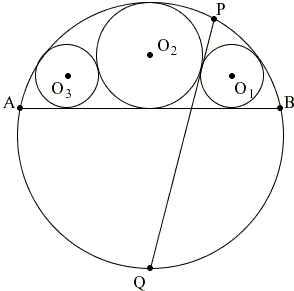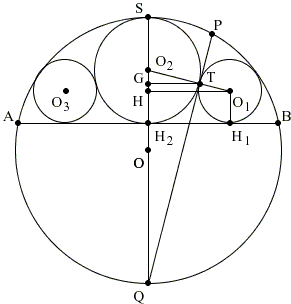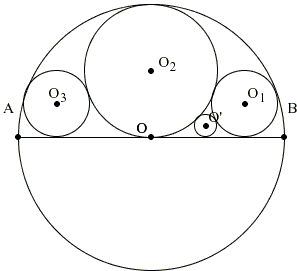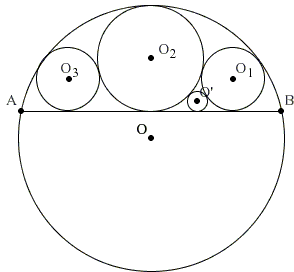# Circles in a Circular Segment

Many a sangaku come in clearly interrelated sequences, although their creation might have been spread of a few decades. We'll start with an example from [Fukagawa and Pedoe, Example 1.6].A chord AB divides a circle O(r) into two segments. A chain of three contact circles O3(r1), O2(r2), O1(r1) all touch AB and also O(r) internally, as shown in the figure. The tangent at the point of contact of the circle O2(r2) and O1(r1) meets the circle O(r) in P and Q. Show that Q is the midpoint of the arc AB, and find the length of PQ in terms r1 and r2.

Solution### References

1. H. Fukagawa, D. Pedoe, Japanese Temple Geometry Problems, The Charles Babbage Research Center, Winnipeg, 1989

Write to:

Charles Babbage Research Center
P.O. Box 272, St. Norbert Postal Station
Winnipeg, MBA chord AB divides a circle O(r) into two segments. A chain of three contact circles O3(r1), O2(r2), O1(r1) all touch AB and also O(r) internally, as shown in the figure. The tangent at the point of contact of the circle O2(r2) and O1(r1) meets the circle O(r) in P and Q. Show that Q is the midpoint of the arc AB, and find the length of PQ in terms r1 and r2.

The tablet has been written in 1843, in the Aichi prefecture, and has since disappeared.

### Solution

We have two show that the common tangent of the two circles O2(r2) and O1(r1) passes through the midpoint of the arc AB and also find the length of that tangent inside the circle O(r). The first task has been accomplished elsewhere by noting that the tangent serves as the radical axis of the two circles. Below we prove this result by computing the lengths of several line segments and using the converse of the Pythagorean theorem to show that ΔQTO2 is right. By the same means we find the length of PQ.With the reference to the diagram above, there are several right triangles to which we may fruitfully apply the Pythagorean theorem:

 ΔO2HO1: (r2 - r1)² + (HO1)² = (r1 + r2)², ΔHOO1: (r - 2r2 + r1)² + (HO1)² = (r - r1)²,

from the first we obtain (HO1)² = 4r1r2 as in a related sangaku.

Substituting this into the second equation we get

r = r2² / (r2 - r1).

Further, the similarity triangles HO1O2 and GTO2

implies the proportion GT / HO1 = TO2 / O1O2, so that

GT = 2r2(r1r2) / (r1 + r2).

From the same triangles, GO2 / HO2 = O2T / O1O2 giving

GO2 = r2(r2 - r1) / (r1 + r2).

Therefore,

GQ = 2r - r2 - r2(r2 - r1) / (r1 + r2).

One more application of the Pythagorean theorem helps determine QT from

QT² = GQ² + GT²

As the last step, we would like to show that ΔQO2T is right (which would imply that QT is indeed tangent to the circles O1(r1) and O2(r2)). To this end, we are in a position to apply the converse of the Pythagorean theorem: suffice it to show that

QT² = O2Q² - O2T² = (r + r2)² - r2²,

which is left as an exercise.

From the orthogonality of QT and O2T we see that triangles QO2T and QSP are similar (∠QPS = 90° as subtended by the diameter QS.) Thus we get an additional proportion:

PQ / QT = QS / O2T,

from which

PQ = QT · QS / O2T = 4r2²(r1r2) / (r2² - r1²).The next two problems are correspondingly [Fukagawa and Pedoe, #1.6.1] and [Fukagawa and Pedoe, #1.6.2]. Both tablets are lost. The first was written in 1857, Ibaragi prefecture, the second in 1806, Nagano prefecture.

The circles are tangent as shown. AB is the diameter of O(r). Find r1, r2 and r' in terms of r.Solution: this is a combination of the above and another sangaku.

r2 = r/2, r1 = r/4, r' = r / (2 + 2)².AB is no longer a diameter; the problem is to express r1, r2 and r' in terms of r and AB.## Sangaku